Courses

# Three Phase Transformer Phase Groups (Part - 1) Electrical Engineering (EE) Notes | EduRev

## Electrical Engineering (EE) : Three Phase Transformer Phase Groups (Part - 1) Electrical Engineering (EE) Notes | EduRev

The document Three Phase Transformer Phase Groups (Part - 1) Electrical Engineering (EE) Notes | EduRev is a part of the Electrical Engineering (EE) Course Electrical Machines.
All you need of Electrical Engineering (EE) at this link: Electrical Engineering (EE)

Three Phase Transformer Phase Groups Part - I
So far, we have seen some constructional features of a 3 phase transformer, and we have indicated that even for the simplest of the 3 phase transformer, there are six windings and twelve terminals.

(Refer Slide Time: 01:12)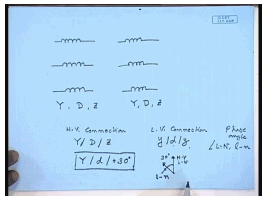So, there are three high voltage windings and three low voltage windings. This is the minimum. In many cases, either the high voltage winding or low voltage winding can be split into two parts giving nine sets of windings. Even in the simplest case, there will be at least six windings. Now, each of this high voltage or low voltage winding can be either star connected or delta connected. And if one set is split into two, it is also possible to have a zigzag connection. So, theoretically, either set of winding – high voltage or low voltage can be star delta or zigzag connected. So, in order to completely specify the transformer winding connection, we have to mention the high voltage connection whether it is star-HV connection, whether it is star-delta or zigzag. Similarly, the LV connection; whether it is star-delta or zigzag. However, that is not sufficient.

In our last lecture, we have seen how to find out the line voltage and current as well as phase voltage and current conversion ratios of a 3 phase transformer, even the winding turns ratio. So, we have seen that, the voltage magnitudes on either sides are different. That is the purpose of the transformer. However, a closest scrutiny will reveal that, not only the magnitudes of the high voltage and low voltage sides are different; in many cases, they are also not in phase.

So, when we specify the winding connection on the high voltage and low voltage sides in addition to specifying whether they are star-delta or zigzag connected, we will have to also specify what is the phase angle between the high voltage line to neutral phasor – line to neutral phasor of high voltage; the angle between line to neutral phasor of high voltage and low voltage winding. So, a complex specification of 3 phase transformer winding connection will involve specifying the high voltage side connection – star-delta or zigzag, low voltage side connection – again star-delta or zigzag, and the phase angle of the low voltage line to neutral voltage phasor with respect to the high voltage line to neutral voltage phasor.

For example, we may specify the connection to be Y, d, plus 30 degree. This means the high voltage is star connected, the high voltage line to neutral phasor – high voltage is star connected; the low voltage is delta connected; and the line to neutral phasor of low voltage winding leads the… This is high voltage line to neutral phasor. This is low voltage line to neutral phasor. This leads the high voltage line to neutral phasor by 30 degree. So, this kind of specification is called the phase group specification of a 3 phase transformer. The phase group specification involves three specifications: one is the connection of the high voltage winding, connection of the low voltage winding and the phase angle between line to neutral voltages of low voltage winding with respect to the high voltage winding.

(Refer Slide Time: 06:29)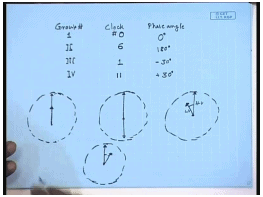Now, this phase angle – there are two conventions of specifying the phase angle. Before we talk about the convention, let us assume what kind of phase angles are possible. There are four types of phase angle that are normally possible. The phase angles can be either 0 degree, that is, the line to neutral voltage phasor of both sides may be cophasor; or, 180 degree, that is, they may be opposite in phase. The one can… The low voltage winding voltage phasor may lag the high voltage winding by 30 degree or it may lead by 30 degree. So, correspondingly, there are four groups. This is called group number 1; this is group number 2; this is group number 3; this is group number 4.

In many cases, particularly, in older installation, this phase angle is indicated by a clock number. For example, if we take the analogy of a clock and say that, the minute hand represents the line to neutral phasor of the high voltage side; and the hour hand, which is smaller represent the line to neutral phasor of the low voltage side; then 0 degree phase angle. This is high voltage line to neutral phasor and this is the low voltage neutral phasor.

On a clock, if we assume this to be a clock, then this is 0 hour. So, this is called 0 – clock number 0. Similarly, for 180 degree, this is the high voltage-voltage phasor; this is the low voltage-voltage phasor; their angle being 180 degree. So, on a clock, this represents 6 o’ clock. So, this is clock number, is 6 for this. For minus 30 degree, this is the minute hand representing the high voltage side line to neutral phasor and this is the low voltage side neutral phasor – line to neutral phasor. This is HV; this is LV. Here the line to neutral phasor of the LV side leads the line to neutral phasor of the HV side by 30 degree. So, this is group number 4. This is called… This copy… This represents 11 o’clock. Therefore, this clock number for plus 30 degree is 11. Similarly, for minus 30 degree, this is HV line to neutral phasor; this is LV line to neutral phasor. This represents 1 o’clock. Therefore, this clock number is 1. Hence, actually, the phase groups can be specified either in terms of phase angle or in terms of clock number.

(Refer Slide Time: 10:39)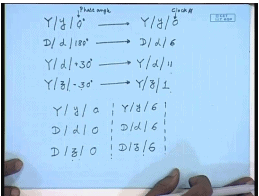Therefore, for example, this Y, y, 0 degree can also be written as Y, y, 0. This is the phase angle. This is clock number. Similarly, D, d, 180 degree can also be written as D, d, 6, because this is the clock number representing 180 degree phase side. Let us say Y, D, plus 30 degree. This will be… Corresponding clock number representation will be Y, d, 11. Let us say Y, z, minus 30 degree. This will be… Corresponding clock number representation will be Y, z, 1. So, this is the correspondence between phase angle representation and clock number representation. They are interchangeable. And in many cases, the phase groups will be specified like this; in many cases, it will be specified like this. But, they represent the same thing.

Let us see what are the different combinations possible. For example, it is easy to understand that, we can always have a Y, y, 0; D, d, 0; or, D, z, 0. In all cases, of course, the high voltage and low voltage side connections may be interchanged. This is one of the possible phase groups – set of phase groups with 0 degree phase angle. We can also have Y, y, 6; D, d, 6 or D, z, 6. However, it is interesting to note that, Y y, Y z 6 or 0 is not possible; why? We will see shortly. These are 0 degree and 180 degree phase shifted phase groups.

(Refer Slide Time: 14:52)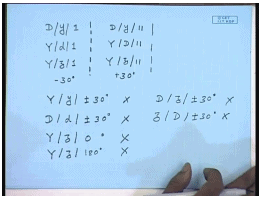For plus minus 30 degrees, you have D, y, 1. This is minus 30 degree. Then… Or, you can have Y, D, 1; or, you can have Y, z, 1. Again, the high voltage and low voltage sides connections can be interchanged. However, D z 1 is not possible. So, this was 0 degree phase shift; this is 180 degree phase shift; this is minus 30 degree phase shift. And then you can have D, y, 11; Y, D, 11; and Y, z, 11.

These are plus 30 degree phase shifted configuration. The configuration that are not possible are Y, y, plus or minus 30 degree – this is not possible; D, d, plus minus 30 degree – this is also not possible; Y, z, 0 degree – not possible; Y, z, 180 degree – not possible; D, z, plus minus 30 degree – this is also not possible; or, z, D, plus minus 30 degree – this is also not possible. So, we have seen that, possibly, there will be twelve different connections of 3 phase transformer. Let us see how these connections are made.

(Refer Slide Time: 17:31)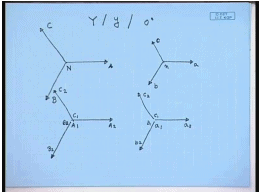Let us start with the simplest one, that is, Y, y, 0 degree. So, how the Y, y, 0 degree line to neutral phase voltages will look like – the phasor diagram? Let us say this is phase A of the high voltage side and this is phase B of the high voltage side; this is phase C of the high voltage side. The phase groups says that, the line to neutral voltage of the low voltage side will be cophasor to the line to neutral voltage of the high voltage side. Therefore, if this is the neutral, then on the low voltage side, this will be phase a; this will be phase b; this will be phase c; this will be the neutral. Now, let us say this A is actually the voltage phasor A 2 A 1. And on the low voltage side, this voltage phasor is between small a 2 and small a 1; this is between B 2 and B 1 and B 2; and this phasor is between C 1 and C 2. This is again between b 1 and b 2; this is between c 1 and c 2.

(Refer Slide Time: 19:47)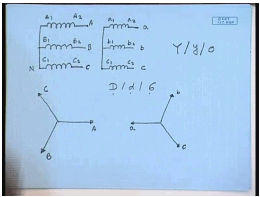Now, from this voltage phasor, it is easy to see how the connections will look like. For example, if these are the windings – high voltage windings; this is A 2 A 1, B 2 B 1, C 2 C 1. And these are the low voltage windings a 2 a 1, b 2 b 1 and c 2 c 1. Then from this phasor diagram, we can see that, the high voltage neutral is formed by joining capital A 1, capital B 1 and capital C 1. So, this will be the neutral point N; this will be the line A; this will be line B; this will be line connected to line C. Similarly, on the low voltage side, the neutral is formed by connecting terminals small a 1, small b 1 and small c 1; and the lines are obtained from the other side. So, this is a, this is b, this is c. So, this is what the connection Y, y, 0 will look like.

Next… So, let us try to do the same thing with a 6 connection; let us say D, d, 6; what does D, d, 6 means? That is the line to neutral phasor of high voltage side and low voltage side should be in phase opposition. So, let us again see. So, this is the line to neutral phasor of the high voltage side A, B, C. Then the low voltage side, we want it to be like this. This we want to be a; this b; and this c. Again let us say; however, please understand that, the connections on the winding are now delta. Therefore, the line to neutral voltage is not same as the voltage across a winding.

(Refer Slide Time: 23:19)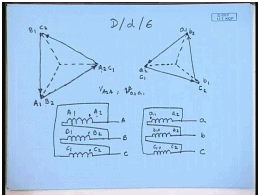However, we still assume that, the lines are connected to A 2; this is terminal A 2; this is terminal B 2; this is terminal C 2. Similarly, on the low voltage side, this is terminal small a 2; this is terminal small b 2; and this is terminal small c 2. Then how do we make the connections? Please remember that, the connections have to be such that, the winding voltages are either in phase or they are in opposite phase. So, what will be the voltages across the windings? It will be the line voltages. So, where will be terminal A 1? So, let us say this is terminal A 1.

So, this is terminal A 1; this is terminal B 1; and this is terminal C 1; that is, the winding voltages are A 1 A 2, B 1 B 2, and C 1 C 2. On the low voltage side, corresponding a 1 a 2 should be in phase with the voltage at… The voltage will be parallel to voltage a 1 a 2; therefore, in phase opposition. So, if we say this terminal is a 1, the phasor a 1 a 2 will be in this direction; if this terminal is c 1, then this is the voltage c 1; and if this terminal is b 1, then this is the voltage b 1. So, we see that, the winding voltages B 1 B 2 and b 1 b 2 are in opposite phase.

Therefore, if we see the winding connections like this with this A 2 A 1, B 2 B 1, C 2 C 1, the delta on the high voltage side is formed by connecting A 1 to B 2, then B 1 to C 2, and C 1 to A 2. And the lines are connected to A 2, B 2 and C 2. These are the lines A, B, C. On the low voltage side also, this is a 1 a 2, b 1 b 2, c 1 c 2. However, the dots are now this way; that is, instead of a 2 and capital A 2 having the same instantaneous voltage, now a 1 and a 2 should have same instantaneous voltage, because we are seeing that, the voltage V A 2 A 1 and the voltage V a 2 a 1 should be in phase opposition. The connections are made again by connecting a 1 to b 2, b 1 to c 2 and c 1 to a 2; and the lines are connected to a 2, b 2, c 2. This is the connection D, d, 6. Similarly, we can see how the connection D, d, 0 or Y, y, 6 will look like.

(Refer Slide Time: 29:49)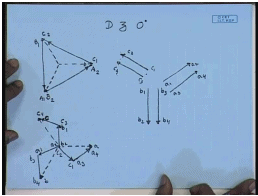So, let us turn our attention to a slightly more complicated connection let us say, D z 0. So, again here we have the line to neutral voltage phasors A, B, C. These are the line to neutral voltage phasors. If we assume the terminals are connected from A 2, B 2 and C 2; then let us say this is A 1. So, the winding voltages A 1 A 2, B 1 B 2 and C 1 C 2. These are the winding voltages. Now, this is the delta side connection A 2, B 2, C 2. Now, it is to be understood that, in a zigzag transformer, each of the secondary winding has two parts.

So, the voltage phasors a 1 a 2… There will be two voltage phasors. This is a 1 a 2 and a 3 a 4, which are parallel to the capital A 1 A 2. Similarly, there will be two voltage phasors: b 1 b 2 and b 3 b 4 and two voltage phasors: c 1 c 2 and c 3 c 4. It is necessary to make the connection as D z 0; which means that, the zigzag side line to neutral voltage should be in phase with the line to neutral voltage of the delta side. Therefore, the zigzag side line to neutral voltage should be… This should be a; this should be b; and this should be c.

Now, in a zigzag transformer, each phase is formed by connecting two different… Each phase is formed by connecting two different winding sections. So, how the… And these two different winding sections belonging to two different phases. So, how can I construct this line to neutral voltage phasor of a zigzag winding using two different phase voltages? One of them will belong to the a phase; the other should be either b phase or c phase. So, it is usually… The last one will be the a phase.

So, let us say this is connected by connecting a 4 a 3. Then the other phase must be – phase voltage must be this. Now, this phase voltage by comparing with this can be either c 2 c 1 or c 3 c 4. Generally, it is the first part that is taking. So, this will be c 1 c 2. Similarly, the b phase will be connected using b 3 b 4 as the last leg; this can be a 1 a 2. c phase will be formed with c 4 c 3 as the last leg; and this will be b 2 b 1.

(Refer Slide Time: 37:20)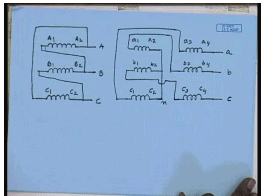So, if we look at the winding connection diagram, this is the high voltage side windings. This is A 2 A 1, B 2 B 1, C 2 C 1. The delta is formed by connecting A 1 to B 2, B 1 to C 2, and C 1 to A 2. These are the lines A, B, C. Now, on the low voltage side, you have two sets of windings here: one set, second set. This is a 1 a 2, a 3 a 4, b 1 b 2, b 3 b 4, c 1 c 2, c 3 c 4. Now, let us look at the phasor diagram. The star is formed by connecting a 2, b 2, c 2. This is the neutral point on the zigzag side. The lines are taken from a 4, b 4, c 4. So, this is terminal a, terminal b and terminal c. Inside we find that, c 1 is connected to a 3. c 1 should be connected to a 3. Similarly, a 1 should be connected to b 3 and b 1 should be connected to c 3. That completes the connection of a D z 0 transformer.

(Refer Slide Time: 41:19)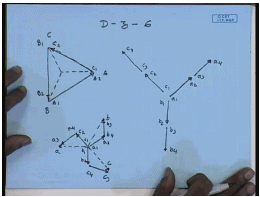How do we get a D – z – 6 degree transformer? D – z – 6 – again the line to neutral voltages are these on the delta side. So, this is the voltage phasor A 1 A 2; this is the voltage phasor C 1 C 2; and this is the voltage phasor B 1 B 2. This is terminal A; this is terminal C; this is terminal B. So, these are the voltage phasors. So, you will have two voltages: a 1 a 2, a 3 a 4; then b 1 b 2, b 3 b 4. These are the different winding voltage phasors that we will obtain on the LV side c 1 c 2, c 3 c 4. Now, the connection is D – z – 6 degree.

So, the line to neutral voltage phasor on the low voltage side will be… This will be a; this will be b; and this will be c. So, the last leg should be formed with a voltage phasor of a and it should point towards this. So, this is found by taking a 3 a 4. So, this is a 3 a 4. Then the next voltage phasor should be this. Obviously, this should be c 2 c 1. Similarly, the b voltage phasor should be formed with b 3 b 4; and this should be a 1 a 2; this should be formed with c 3 c 4; and this should be formed with b 1 b 2.

(Refer Slide Time: 46:10)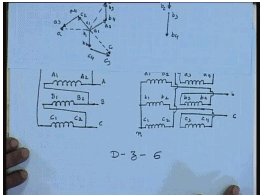Hence, the winding connection wise, this is A 1 A 2, B 1 B 2, C 1 C 2. The connection is A 1 connected to B 2, B 1 connected to C 2, and C 1 connected to A 2. And the supply is given to A 2, B 2, C 2. On the zigzag side, you have two sets of windings: a 1 a 2, a 3 a 4; b 1 b 2, b 3 b 4; c 1 c 2, c 3 c 4. According to the phasor diagram, in this case, the star point is formed by connecting a 1, b 1, c 1. So, this is the star point. And terminals are taken out from a 3, b 3, c 3. So, these are the terminals taken out: a, b and c. Connection wise, a 2 is connected to b 4, b 2 is connected to c 4, and c 2 is connected to a 4. So, this completes the connection of D – z – 6. In the similar manner, remaining connections can be easily drawn.

(Refer Slide Time: 49:40)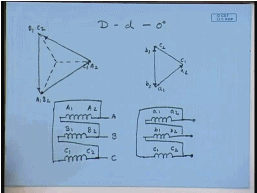Let us say what will be D – d – 0; D d 0 – A, B, C; A 2, B 2, C 2. This is C 1 also; this is A 1; and this is B 1. So, this is the winding voltage phasor A 1, A 2; this is B 1 B 2; and this is C 1 C 2. D – d – 0 means line to neutral phasors are cophasors; so will be the line to line phasors. So, on the secondary side, this will be the phasor; this will be another phasor; and this will be another phasor. This will be a 1 a 2; this will be c 1 c 2; this will be b 1 b 2. Connection wise the simple; this is A 1 A 2, B 1 B 2, C 1 C 2. Connections are made by connecting A 1 to B 2, B 1 to C 2 and C 1 to A 2. And the supply is given to A 2, B 2, C 2.

On the low voltage side, a 1 a 2, b 1 b 2, c 1 c 2. The connection is made by connecting a 1 to b 2, b 1 to c 2, and c 1 to a 2. This case gives the connection of D – d – 0. Please compare this with the connection of D – d – 6. Let us compare it with the connection of D – d – 6. Here also the connection was similar, but here the polarity of the terminals – instantaneous polarity were differs. So, if this has to be reconnected into D – d – 6, these connections are to be reversed. In other words, a 2 has to be connected to b 1, b 2 has to be connected to c 1, and c 2 has to be connected to a 1; and the supply is given to a 1, b 1, c 1. That will give a connection of D – d – 6. Similarly, we can obtain the connection of Y – y – 6; which is left as an exercise.

Thank you.

Offer running on EduRev: Apply code STAYHOME200 to get INR 200 off on our premium plan EduRev Infinity!

,

,

,

,

,

,

,

,

,

,

,

,

,

,

,

,

,

,

,

,

,

;